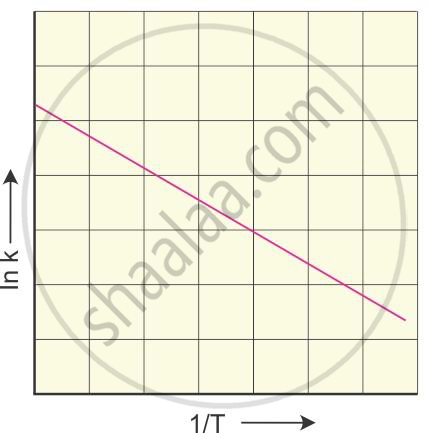HSC Science (General) 12th Board ExamMaharashtra State Board
Share

Explain a graphical method to determine activation energy of a reaction - HSC Science (General) 12th Board Exam - Chemistry

ConceptTemperature Dependence of the Rate of a Reaction

Question

Explain a graphical method to determine activation energy of a reaction.

Solution

The Arrhenius equation is given by

k=Ae^((-E_a)/(RT))

On taking logarithms of both sides, we get

log_nk=log_nA-E_a/(RT)

or

log_10k=-E_a/(2.303RT)+log_10A

log_10k=-E_a/(2.303R) times (1/T)+log_10A

The rate constant of a reaction is determined at various temperatures.

log10 k is plotted against the reciprocal of temperature. The graphical
representation isThe slope of the straight line graph is

–(Ea)/(2.303R)

from which the activation energy can be calculated.

Is there an error in this question or solution?

APPEARS IN

2015-2016 (March) (with solutions)
Question 1.4 | 2.00 marks

Video TutorialsVIEW ALL 

Solution Explain a graphical method to determine activation energy of a reaction Concept: Temperature Dependence of the Rate of a Reaction.
S